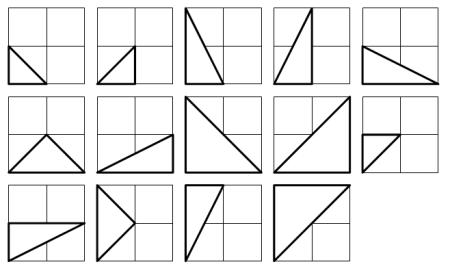# Find all integer sided triangles whose area and peremeter are equalDetermine all triangles whose sides are integers, and the areas and perimeters are (numerically) equal. What is the sum of the longest sides of each of the triangles?

Extra Credit for proving that those are the only solutions. (Put your proof in the solutions section)

Explicit Example: If the triangles are (7, 8, 9) and (13, 5, 9). Then enter your solution as 9+13 = 22

×